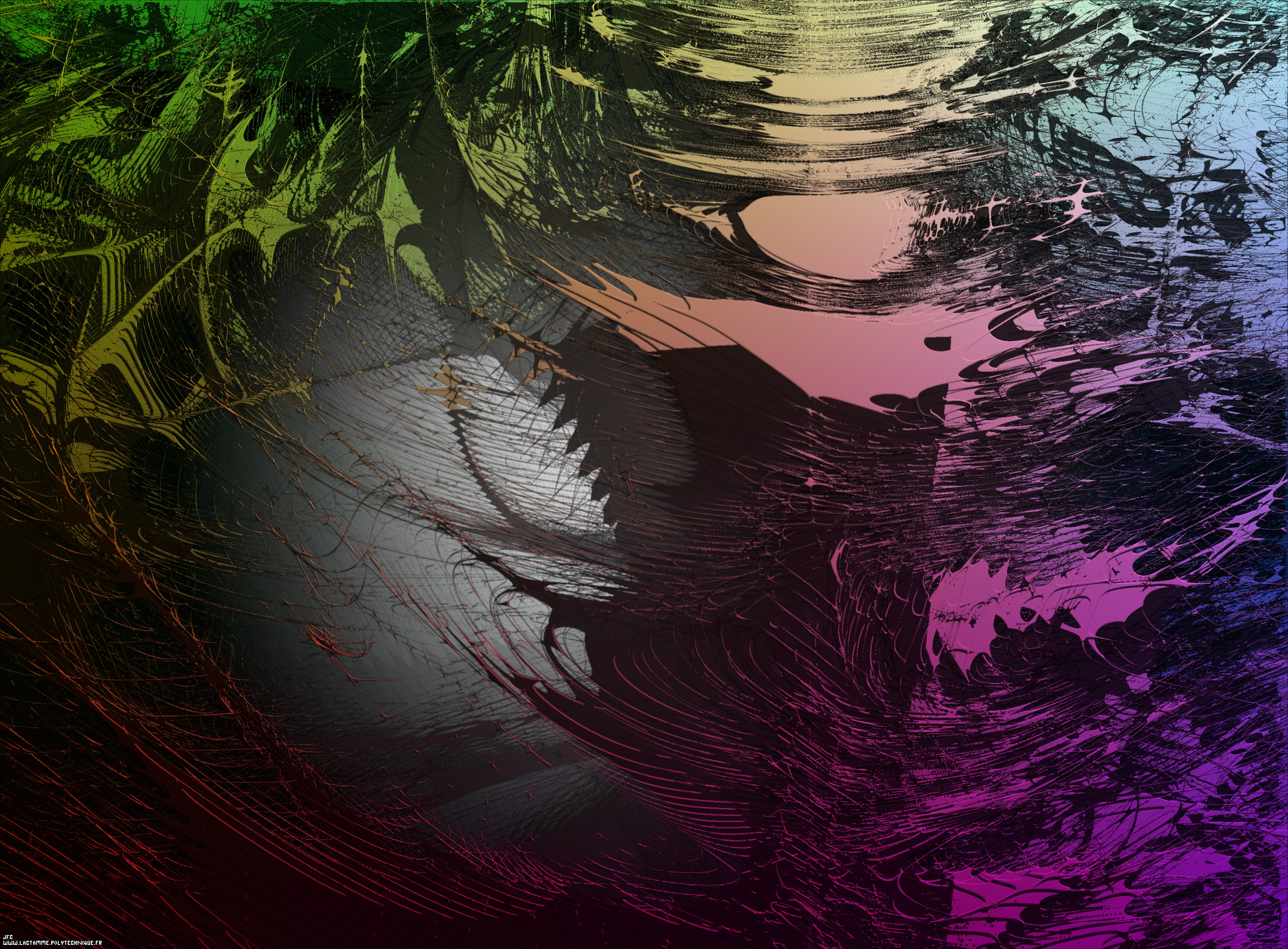Tridimensional visualization of the Verhulst dynamics -'Time Ships', a tribute to Stephen Baxter- [Visualisation tridimensionnelle de la dynamique de Verhulst -'Les Vaisseaux du Temps', un hommage à Stephen Baxter-].

The Verhulst dynamics is defined using the following iteration:
```                    X  = 0.5
0
```
```                    X  = RX   (1 - X   )
n     n-1      n-1
```
Here, in this computation, the growing rate 'R' is no longer constant but changes its value periodically using the following arbitrary cycle:
```R5 ==> R5 ==> R5 ==> R5 ==> R5 ==> R5 ==> R5 ==> R4 ==> R4 ==> R4 ==> R4 ==> R3 ==> R3 ==> R2 ==> R2 ==> R1 ==> R1 ==> R1 ==> R1 ==> R1 ==> R1 ==> R2 ==> R2 ==> R3 ==> R3 ==> R4 ==> R4 ==> R5 ==> R5 ==> R4 ==> R4 ==> R3 ==> R2 ==> R2 ==> R1 ==> R1 ==> R1 ==> R1 ==> R1 ==> R2 ==> R3 ==> R3 ==> R4 ==> R4 ==> R5 ==> R5 ==> R5 ==> R4 ==> R4 ==> R4 ==> R3 ==> R3 ==> R2 ==> R2 ==> R1 ==> R1 ==> R1 ==> R1 ==> R1 ==> R1 ==> R1 ==> R1 ==> R1 ==> R1
```
where {R1,R2,R3,R4,R5} are respectively the five coordinates of the current point inside the following domain [2.936,3.413]x[3.500,3.850]x[3.000,4.000]x[2.7]x[2.9]. Only the points corresponding to a dynamical system with a negative Lyapunov exponent are displayed.

See some related pictures (possibly including this one):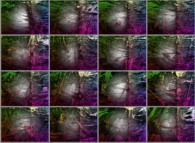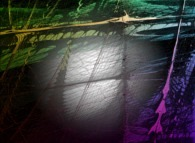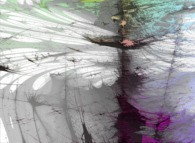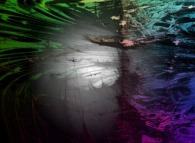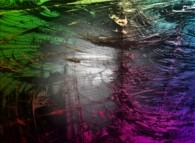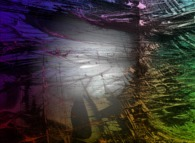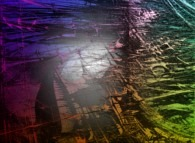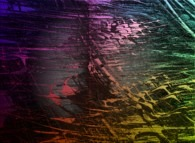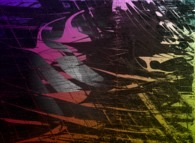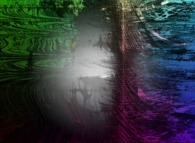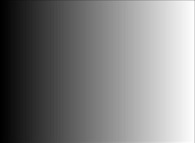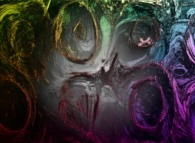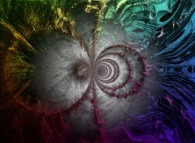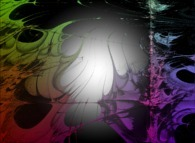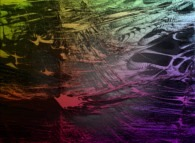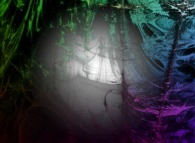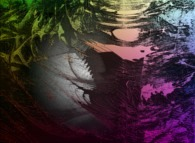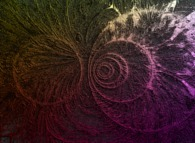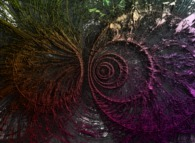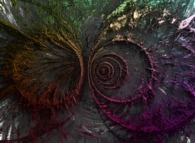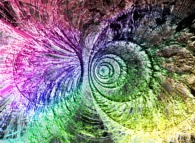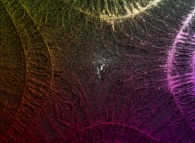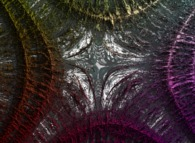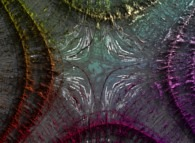See a bidimensionnal dynamics: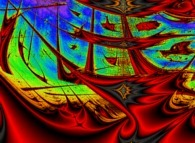(CMAP28 WWW site: this page was created on 10/06/2015 and last updated on 08/25/2019 10:57:25 -CEST-)

[See all related pictures (including this one) [Voir toutes les images associées (incluant celle-ci)]]

[Please visit the related ArtAndScience picture gallery [Visitez la galerie d'images ArtAndScience associée]]
[Please visit the related DeterministicChaos picture gallery [Visitez la galerie d'images DeterministicChaos associée]]
[Please visit the related DeterministicFractalGeometry picture gallery [Visitez la galerie d'images DeterministicFractalGeometry associée]]
[Go back to AVirtualSpaceTimeTravelMachine [Retour à AVirtualSpaceTimeTravelMachine]]
[The Y2K bug [Le bug de l'an 2000]]

[Site Map, Help and Search [Plan du Site, Aide et Recherche]]
[Mail [Courrier]]
[About Pictures and Animations [A Propos des Images et des Animations]]